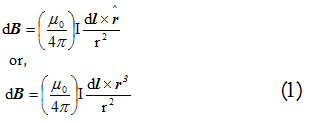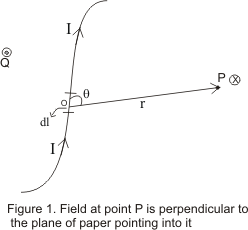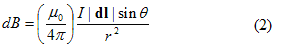Magnetic Effect of current|Biot Savart Law

(1) Introduction

• In the previous chapter we have defined concept of magnetic field represented by vector B
• We defined magnetic field B in terms of force it exerts on moving charges and on current carrying conductors
• We also know that magnetic field is produced by the motion of the electric charges or electric current
• In this chapter we would study the magnetic field produced by the steady current
• we would study about how various factors affect the magnitude and direction of the magnetic field
• We will also learn to calculate the equation for magnetic field B if the current configuration is known using Biot-savart's law and ampere circuital law

(2)Biot Savart Law

• We know that electric current or moving charges are source of magnetic field
• A Small current carrying conductor of length dl (length element ) carrying current I is a elementary source of magnetic field .The force on another similar conductor can be expressed conveniently in terms of magnetic field dB due to the first
• The dependence of magnetic field dB on current I ,on size and orientation of the length element dl and on distance r was first guessed by Biot and savart
• The magnitude of the magnetic field dB at a distance r from a current element dl carrying current I is found to be proportional to I ,to the length dl and inversely proportional to the square of the distance |r|
• The direction of the magnetic Field is perpendicular to the line element dl as well as radius r
• Mathematically, Field dB is written asHere (μ0/4π) is the proportionality constant such that
μ0/4π=10-7 Tesla Meter/Ampere(Tm/A)
• Figure below illustrates the relation between magnetic field and current element• if in figure, Consider that line element dl and radius vector r connecting line element mid point to the field point P at which field is to be found are in the plane of the paper
• From equation (1) ,we expect magnetic field to be perpendicular to both dl and r.Thus direction of dB is the direction of advance of right hand screw whose axis is perpendicular to the plane formed by dl and r and which is rotated from dl to r ( right hand screw rule of vector product)
• Thus in figure ,dB at point P is perpendicular directed downwards represented by the symbol (x) and point Q field is directed in upward direction represented by the symbol (•)
• The magnitude of magnetic field iswhere θ is the angle between the line element dl and radius vector r
• The resultant field at point P due to whole conductor can be found by integrating equation (1) over the length of the conductor i.e.
B=∫dB

Relation between permeability (μ0 and permittivity (ε0) of the free space
• We know that
μ0/4π=10-7 N/A2 ----(a)
and
1/4πε0=9*109 N-m2/C2 ----(b)
Dividing equation a by b we get
μ0ε0 =1/(9*1016) (C/Am)2
we know that
1C=1A-s

So μ0ε0 =1/(3*108 m/s)2
And 3*108 m/s is the speed of the light in free space
So μ0ε0 =1/c2
or c=1/√(μ0ε0)

Note to our visitors :-

Thanks for visiting our website.
DISCLOSURE: THIS PAGE MAY CONTAIN AFFILIATE LINKS, MEANING I GET A COMMISSION IF YOU DECIDE TO MAKE A PURCHASE THROUGH MY LINKS, AT NO COST TO YOU. PLEASE READ MY DISCLOSURE FOR MORE INFO.